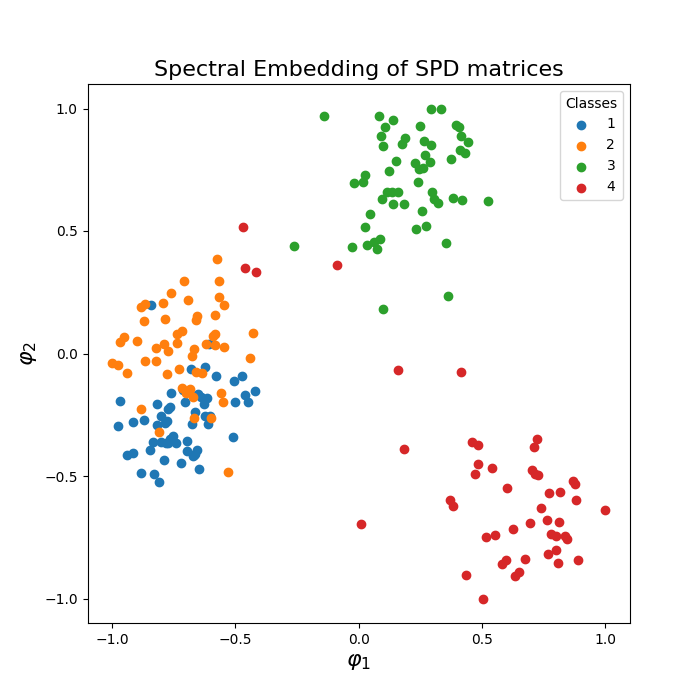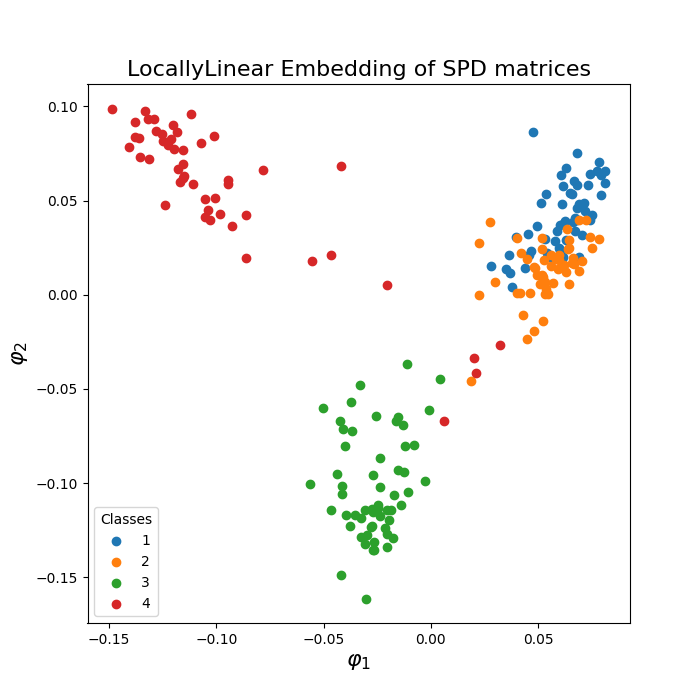# Embedding ERP MEG data in 2D Euclidean space¶

Riemannian embeddings via Laplacian Eigenmaps (LE) and Locally Linear Embedding (LLE) of a set of ERP data. Embedding via Laplacian Eigenmaps is referred to as Spectral Embedding (SE).

Locally Linear Embedding (LLE) assumes that the local neighborhood of a point on the manifold can be well approximated by the affine subspace spanned by the k-nearest neighbors of the point and finds a low-dimensional embedding of the data based on these affine approximations.

Laplacian Eigenmaps (LE) are based on computing the low dimensional representation that best preserves locality instead of local linearity in LLE .

```# Authors:  Pedro Rodrigues <pedro.rodrigues01@gmail.com>,
#           Gabriel Wagner vom Berg <gabriel@bccn-berlin.de>

from pyriemann.estimation import XdawnCovariances
from pyriemann.utils.viz import plot_embedding

import mne
from mne import io
from mne.datasets import sample

from sklearn.model_selection import train_test_split

import matplotlib.pyplot as plt

print(__doc__)
```

```data_path = str(sample.data_path())
raw_fname = data_path + '/MEG/sample/sample_audvis_filt-0-40_raw.fif'
event_fname = data_path + '/MEG/sample/sample_audvis_filt-0-40_raw-eve.fif'
tmin, tmax = -0., 1
event_id = dict(aud_l=1, aud_r=2, vis_l=3, vis_r=4)

# Setup for reading the raw data
raw.filter(2, None, method='iir')  # replace baselining with high-pass

picks = mne.pick_types(raw.info, meg=True, eeg=False, stim=False, eog=False,

epochs = mne.Epochs(raw, events, event_id, tmin, tmax, proj=False,

X = epochs.get_data()
y = epochs.events[:, -1]
```
```Filtering raw data in 1 contiguous segment
Setting up high-pass filter at 2 Hz

IIR filter parameters
---------------------
Butterworth highpass zero-phase (two-pass forward and reverse) non-causal filter:
- Filter order 8 (effective, after forward-backward)
- Cutoff at 2.00 Hz: -6.02 dB
```

Embedding of Xdawn covariance matrices

```nfilter = 4
xdwn = XdawnCovariances(estimator='scm', nfilter=nfilter)
split = train_test_split(X, y, train_size=0.25, random_state=42)
Xtrain, Xtest, ytrain, ytest = split
covs = xdwn.fit(Xtrain, ytrain).transform(Xtest)
```

## Laplacian Eigenmaps (LE), also called Spectral Embedding (SE)¶

```plot_embedding(covs, ytest, metric='riemann', embd_type='Spectral',
normalize=True)
plt.show()
```## Locally Linear Embedding (LLE)¶

```plot_embedding(covs, ytest, metric='riemann', embd_type='LocallyLinear',
normalize=False)
plt.show()
```